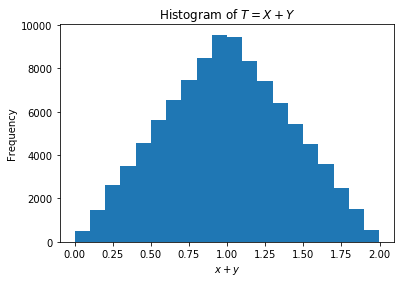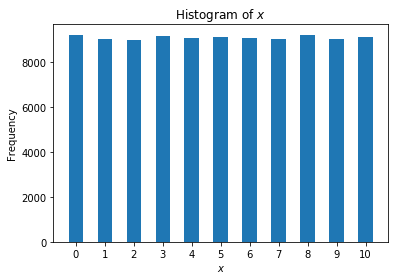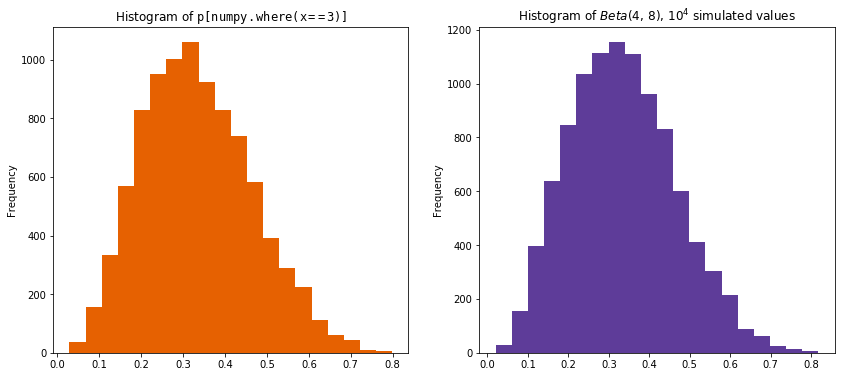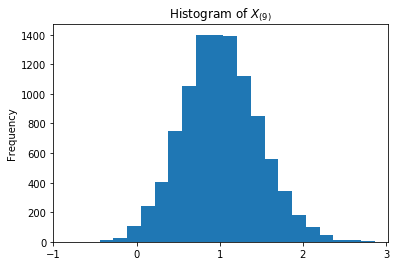# Chapter 8: Transformations¶

This Jupyter notebook is the Python equivalent of the R code in section 8.8 R, pp. 373 - 375, Introduction to Probability, 1st Edition, Blitzstein & Hwang.

In :
import matplotlib.pyplot as plt
import numpy as np

%matplotlib inline


## Beta and Gamma distributions¶

In :
from scipy.stats import beta, gamma

# to learn more about scipy.stats.beta, un-comment ouf the following line
#print(beta.__doc__)

# to learn more about scipy.stats.gamma, un-comment ouf the following line
#rint(gamma.__doc__)


The Beta and Gamma distributions are implemented in scipy.stats.beta and scipy.stats.gamma, respectively.

• To evaluate the $Beta(a, b)$ PDF or CDF at $x$, we use beta.pdf(x, a, b) and beta.cdf(x, a, b). To generate n realizations from the $Beta(a, b)$ distribution, we use beta.rvs(a, b, size=n).
• To evaluate the $Gamma(a, \lambda)$ PDF or CDF at $x$, we usegamma.pdf(x, a, scale=1/lambd) or gamma.cdf(x, a, scale=1/lambd). To generate $n$ realizations from the $Gamma(a, \lambda)$ distribution, we use gamma.rvs(a, scale=1/lambd, size=n).
• The $\lambda$ parameter in $Gamma(a, \lambda)$ corresponds in gamma to using scale = $\frac{1}{\lambda}$ and default value of loc = 0.

For example, we can check that the $Gamma(3, 2)$ distribution has mean $\frac{3}{2}$ and variance $\frac{3}{4}$. To do this, we generate a large number of $Gamma(3, 2)$ random variables using gamma.rvs, then compute their mean and var using their corresponding methods in numpy.array (you could of course also use numpy.mean and numpy.var, passing in the array of r.v.):

In :
# seed the random number generator
np.random.seed(317811)

alpha = 3.0
lambd = 2.0

y = gamma.rvs(alpha, scale=1/lambd, size=10**5)

mean = y.mean()
#mean = np.mean(y)
print('mean of Gamma(3, 2) = {}'.format(mean))

var = y.var()
#var = np.var(y)
print('variance of Gamma(3, 2) = {}'.format(var))

mean of Gamma(3, 2) = 1.5012880719327166
variance of Gamma(3, 2) = 0.7512319256875035


NOTE: lambda in Python is a reserved keyword for declaring small, anonymous functions, and so we cannot used the name lambda for variable or functions.

Try changing the numpy.random.seed input value in the code block above, and hit SHIFT+ENTER to re-execute the code block. Did you get values that are close to 1.5 and 0.75, respectively?

## Convolution of Uniforms¶

Using scipy.stats.uniform and matplotlib.pyplot.hist, we can quickly verify that for $X, Y \stackrel{i.i.d.}{\sim} Unif(0, 1)$, the distribution of $T = X + Y$ is triangular in shape:

In :
np.random.seed(514229)

from scipy.stats import uniform

x = uniform.rvs(size=10**5)
y = uniform.rvs(size=10**5)

t = x + y

plt.hist(t, bins=20)

plt.title(r'Histogram of $T = X + Y$')
plt.xlabel(r'$x + y$')
plt.ylabel('Frequency')

plt.show()The histogram looks like an ascending and then descending staircase, a discrete approximation to a triangle.

## Bayes' billiards¶

In the Bayes' billiards story, we have $n$ white balls and 1 gray ball, throw them onto the unit interval completely at random, and count the number of white balls to the left of the gray ball. Letting $p$ be the position of the gray ball and $X$ be the number of white balls to the left of the gray ball, we have

\begin{align} p &\sim Unif(0, 1) \\ X|p &\sim Bin(n, p) \end{align}

By performing this experiment a large number of times, we can verify the results we derived in this chapter about the marginal PMF of $X$ and the posterior PDF of $p \text{ given } X = x$. We'll let the number of simulations be called nsims, to avoid a name conflict with the number of white balls, n, which we set equal to 10:

In :
nsims = 10**5
n = 10


We simulate 105 values of $p$, then simulate 105 values from the conditional distribution of $X$ given $p$:

In :
np.random.seed(832040)

p = uniform.rvs(size=nsims)

from scipy.stats import binom

x = binom.rvs(n, p, size=nsims)


Notice that we feed the entire array p into binom.rvs. This means that the first element of x is generated using the first element of p, the second element of x is generated using the second element of p, and so forth. Thus, conditional on a particular element of p, the corresponding element of x is Binomial, but the elements of p are themselves Uniform, exactly as the model specifies.

According to the Bayes' billiards argument, the marginal distribution of $X$ should be Discrete Uniform on the integers 0 through $n$. Is this in fact the case? We can make a histogram of x to check! Because the distribution of $X$ is discrete, we can specify bins=np.arange(0, n+1, 0.5), align='left' in the call to matplotlib.pyplot.hist so that each bar is centered at an integer value:

In :
plt.hist(x, bins=np.arange(0, n+1, 0.5), align='left')

plt.title(r'Histogram of $x$')
plt.xlabel(r'$x$')
plt.ylabel('Frequency')
# also set the x-axis ticks to show integers 0, 1, 2, ..., 10
plt.xticks(range(0, 10+1))

plt.show()Indeed, all the histogram bars are approximately equal in height, consistent with a Discrete Uniform distribution.

Now for the posterior distribution of $p$ given $X = x$. Conditioning is very simple since we are using numpy.array. To consider only the simulated values of $p$ where the value of $X$ was 3, we use numpy.where(x==3) to find the indices where x = 3, and then use that within the square brackets of p to index only those corresponding values. In particular, we can create a histogram of these values using matplotlib.pyplot.hist to see what the posterior distribution of $p$ given $X = 3$ looks like.

According to the Beta-Binomial conjugacy result, the true posterior distribution is $p|X = 3 \sim Beta(4, 8)$. We can plot the histogram of p[numpy.where(x==3)] next to a histogram of simulated values from the $Beta(4, 8)$ distribution to confirm that they look similar:

In :
np.random.seed(1346269)

fig = plt.figure(figsize=(14, 6))

p_given_x_3 = p[np.where(x==3)]

ax1.hist(p_given_x_3, bins=20, color='#e66101')
ax1.set_title(r'Histogram of $\tt{p[numpy.where(x==3)]}$')
ax1.set_ylabel('Frequency')

brv = beta.rvs(4.0, 8.0, size=10**4)

ax2.hist(brv, bins=20, color='#5e3c99')
ax2.set_title(r'Histogram of $Beta(4, \, 8)$, $10^4$ simulated values')
ax2.set_ylabel('Frequency')

plt.show()For the side-by-side graph above, we obtain an instance of matplotlib.pyplot.figure from pyplot, specifying the figure's width and height. Next, we use figure.add_subplot(121) to create graph axes in a figure with 1 row and 2 columns, in the 1st column position from the left, for the histogram of p[numpy.where(x==3)]. Likewise, figure.add_subplot(122) creates the graph axes for the histogram for $Beta(4, 8)$ in the 2nd column position of the 1-row, 2-column figure.

## Simulating order statistics¶

Simulating order statistics in Python/NumPy/SciPy is easy: we simply simulate i.i.d. r.v.s and sort them in order. For example,

In :
np.random.seed(2178309)

from scipy.stats import norm

rv = np.sort(norm.rvs(size=10))
print(rv)

[-2.05800632 -1.14300612 -0.09108752 -0.08188422  0.45810851  0.55556877
0.59398037  0.9312065   0.98367818  1.12202827]


produces one realization of $X_{(1)}, \ldots, X_{(10)}$, where $X_{(1)}, \ldots, X_{(10)}$ are i.i.d. $N(0, 1)$. If we want to plot a histogram of realizations of, say, $X_{(9)}$, we'll need to use iteration. Let's try using Python's list comprehensions:

In :
np.random.seed(3524578)

rv_arr = [np.sort(norm.rvs(size=10)) for _ in range(10**4)]
order_stats = np.array(rv_arr)

print('order_stats has shape: {}'.format(order_stats.shape))

order_stats has shape: (10000, 10)


The 104 iterations are done with

rv_arr = [np.sort(norm.rvs(size=10)) for _ in range(10**4)]



This Python list comprehension does the following:

• loops 104 times with for _ in range(10**4), where we ignore the iteration counter by using _
• as we loop, we do not need to know which particular iteration we are on
• for each iteration, calculate np.sort(norm.rvs(size=10))
• gather up the results of all the iterations into a Python list (array), assigning this resulting list to rv_arr
• this one-liner list comprehension is equivalent to all this code:
  rv_arr = []
for _ in range(10**4):
rv = np.sort(norm.rvs(size=10))
rv_arr.append(rv)


This creates a matrix, order_stats, with 104 rows and 10 columns. The ith column of the matrix contains 104 realizations of $X_{(i+1)}$ (don't forget that Python is zero-indexed, so the 8th column is $X_{(9)}$!). Now we can create a histogram of $X_{(9)}$, simply by selecting column 8 of the matrix:

In :
x9 = order_stats[:, 8]

plt.hist(x9, bins=20)

plt.xticks(np.arange(-1, 4, 1))
plt.title(r'Histogram of $X_{(9)}$')
plt.ylabel('Frequency')

plt.show()We can also compute summaries like numpy.mean(x9) and numpy.var(x9).

In :
mean_x9 = np.mean(x9)
print('mean of x9 = {}'.format(mean_x9))

var_x9 = np.var(x9)
print('variance of x9 = {}'.format(var_x9))

mean of x9 = 1.0092010380841254
variance of x9 = 0.2108867888683199


© Blitzstein, Joseph K.; Hwang, Jessica. Introduction to Probability (Chapman & Hall/CRC Texts in Statistical Science).